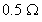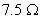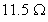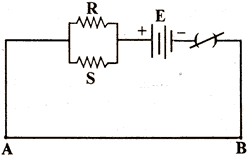Friday 10th July 2020

CBSE Guess > Papers > Question Papers > Class XII > 2004 > Physics > Compartment Outside Delhi Set-II

PHYSICS (Set II— Compartment Outside Delhi)

Except for the following questions, all the remaining questions have been asked in Set I.

Q. 1. Write the full forms of the terms (i) MSI and (ii) LSI used for different types of integrated circuits. 1

Q. 4. Name the type of communication that uses carrier signals having frequenciesin the range 1012 Hz or 1016 Hz.

Q. 5. Two identical coherent waves, each of intensity I, are producing an interference pattern. Write the value of the resultant intensity at a point of (i) constructive interference and (ii) destructive interference 1

Q. 7. A cell, of emf 4 V and internal resistance. is connected across a load of resistance (i), (ii)Calculate (i) the ratio of the differences in the emf of the cell and the potential drop across the load, and (ii) the ratio of the currents in the two cases. 2

Q. 8. Name and define the unit used for measuring the coefficient of mutual inductance. State the relation of this unit with the units of magnetic flux and electric current. 2

Q. 11. A logic gate has been obtained by applying the negation (NOT) operation after OR gate. Name the gate so formed. Write the symbol and the truth table of this gate. 3

Q. 13. A potentiometer wire has a length L and a resistance R0. It is connected to a battery and a resistance combination as shown. Obtain an expression for the potential drop per unit length of this potentiometer wire.
What is the maximum emf of a ‘test cell’ for which one can get a ‘balance point’ on this potentiometer wire? What precaution should one take, while connecting this ‘test cell’, in the circuit? 3Q. 15. When can a charge act as a source of electromagnetic waves? How are the directions, of the electric and magnetic field vectors, in an electromagnetic wave, related to each other and to the direction of propagation of the wave?
Which physical quantity, if any, has the same value for waves belonging to the different parts of the electromagnetic spectrum? 3

Q. 18. Name the three types of magnetic materials which behave differently when placed in a non uniform magnetic field. Give two properties for each of them.3

Q. 24. The half-life, of a given radioactive nuclide, is 138.6 days. What is the mean life of this nuclide? After how much time will a given sample of this radioactive nuclide get reduced to only 12.5% of its initial value? 3

 Physics 2004 Question Papers Class XII Delhi Outside Delhi Compartment Delhi Compartment Outside DelhiSet ISet ISet ISet ISet IISet IISet IISet IISet IIISet III

CBSE 2004 Question Papers Class XII